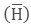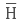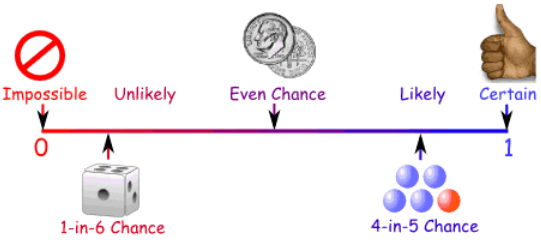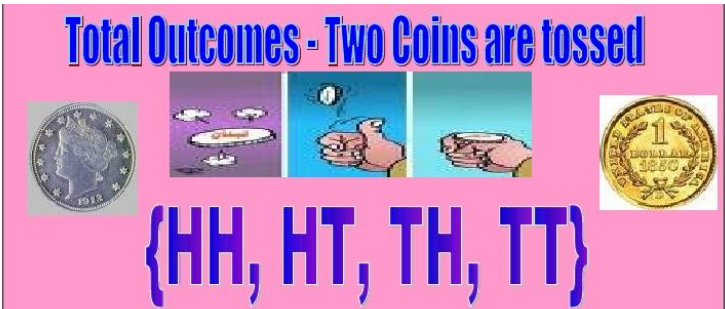×#### Thank you for registering.

One of our academic counsellors will contact you within 1 working day.

Click to Chat

1800-1023-196

+91-120-4616500

CART 0

• 0

MY CART (5)

Use Coupon: CART20 and get 20% off on all online Study Material

ITEM
DETAILS
MRP
DISCOUNT
FINAL PRICE
Total Price: Rs.

There are no items in this cart.
Continue Shopping• Complete JEE Main/Advanced Course and Test Series
• OFFERED PRICE: Rs. 15,900
• View Details

```Revision Notes on Probability

Probability

Probability is the study of mathematics which calculates the degree of uncertainty. There are two types of approaches to study probability-

1. Experimental or Empirical Probability

The result of probability based on the actual experiment is called experimental probability. In this case, the results could be different if we do the same experiment again.2. Probability — A Theoretical Approach

In the theoretical approach, we predict the results without performing the experiment actually. The other name of theoretical probability is classical probability.Where the outcomes are equally likely.

Equally Likely Outcomes

If we have the same possibility of getting each outcome then it is called equally likely outcomes.

Example

A dice have the same possibility of getting 1, 2, 3, 4, 5 and 6.

Not Equally Likely

If we don't have the same possibility of getting each outcome then it is said to be the not equally likely outcome.

Example

3 green balls and 2 pink balls are not equally likely as the possibility of the green ball is 3 and the possibility of the pink ball is 2.

Elementary Event

If an event has only one possible outcome then it is called an elementary event.

Remark

The sum of the probabilities of all the elementary events of an experiment is 1.

The General form

P (Heads) + P (Tails) = 1

P (H) + P= 1 whereis ‘not H’.

P (H) – 1 = PP (H) and Pare the complementary events.

Impossible Events

If there is no possibility of an event to occur then its probability is zero. This is known as an impossible event.

Example

It is not possible to draw a green ball from a group of blue balls.

Sure or Certain Event

If the possibility of an event to occur is sure then it is said to be the sure probability. Here the probability is one.

This shows that the probability of an event could be

0 ≤ P (E) ≤ 1Some Solved Examples

Example: 1

What is the probability of drawing a heart from a deck of cards?

Solution:

We know that there are total 52 cards in a deck out of which 13 cards are of heart.

So the favourable outcomes are 13 and the total no. of events is 52.= 13/52 = 1/4

Example: 2

If we toss two coins together, then what is the probability of getting at least one tail?

Solution:

If we toss two coins together then the total outcomes could beThe favorable outcomes for at least one head will be

{HH}, {HT}, {TH} = 3

P (for at least one head) = 3/4
```### Course Features

• 728 Video Lectures
• Revision Notes
• Previous Year Papers
• Mind Map
• Study Planner
• NCERT Solutions
• Discussion Forum
• Test paper with Video Solution